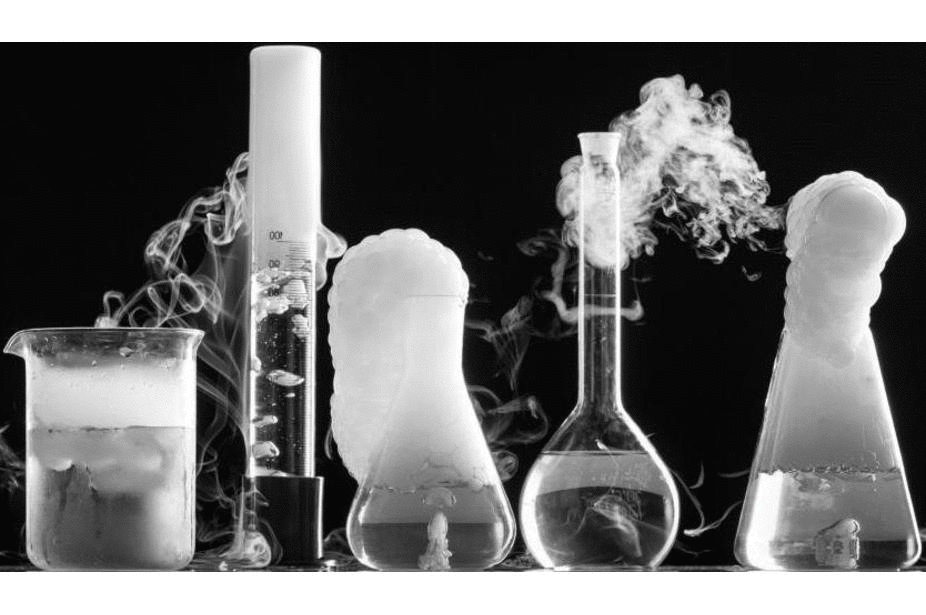## Types of Chemical Reactions

Chemical reactions are reactions which involve making and breaking of bonds between atom to yield new substances.

### 1.    Combination Reaction:

These are the reactions in which one single product is formed from two or more reactants.
Example: i. Formation of Calcium Hydroxide.
CaO (s)(quick lime) + H2O (l) ----> Ca(OH)2 (aq)(slaked lime)
ii. Burning of Coal.
C (s) + O2 (g) ----> CO2 (g)
iii. Formation of Water.
2H2 (g) + O2 (g) ----> 2H2O (l)

### 2.    Displacement Reaction:

These are the reactions in which highly reactive metal displaces less reactive metal from their solution.
Example: i. Fe (s) + CuSO4 (aq)(copper sulphate) ----> FeSO4 (aq)(iron sulphate) + Cu (s)
ii. Zn (s) + CuSO4 (aq)(copper sulphate) ------> ZnSO4 (aq)(zinc sulphate) + Cu (s)
iii. Pb (s) + CuCl2 (aq)(copper chloride) ------> PbCl2 (aq)(lead chloride) + Cu (s)

### 3.    Double Displacement Reaction:

These are the reactions in which exchange of ions between reactants occur.
Example: Na­2SO4 (aq)(sodium sulphate) + BaCl2 (aq)(barium chloride) -----> BaSO4 (s)(barium sulphate) + 2NaCl (aq)(sodium chloride)

### 4.    Oxidation and Reduction Reaction:

#### 1.1.        Oxidation:

These are the reactions in which a substance loses hydrogen or gain oxygen.
Example: 2Cu + O2 + Heat ---------> 2CuO

#### 1.2.        Reduction:

These are the reactions in which a substance gain hydrogen or loses oxygen.
Example: CuO + H2 + Heat -------> Cu + H2O

#### 1.3.        Redox Reaction:

These are the reactions in which one reactant gets reduced while other reactant gets oxidized. This type of reaction is also known as Oxidation-Reduction Reaction or Redox Reaction.
Example:
i.                   CuO + H2 + Heat ------> Cu + H2O
In above reaction-
H2 ------> H2O (oxidation)

CuO -----> Cu (reduction)
ii.                ZnO + C ------>  Zn + CO
iii.             MnO2 + 4HCl ------> MnCl2 + 2H2O + Cl2

### Observable Effect of Oxidation Reduction in Everyday Life:

#### 1.    Corrosion:

Attack on metals by moisture, acids, oxygen etc., which corrode metals is called corrosion.

Example: Rusting of Iron, Black coating on Silver, Green coating on Copper.

#### 2.    Rancidity:

Change in taste and smell of food material prepared by using fat or oil, because fat or oil get oxidized and become rancid.

### Endothermic Reaction:

These are the reactions in which heat is absorbed during reaction.

Example: NH4Cl (s) + H2O (l) + Heat -                    -------> NH4Cl (aq)

### Exothermic Reaction:

These are the reactions in which heat is evolved during reaction.

Example: CH4 (g) + 2O2 (g) -----> CO2 (g) + 2H2O (g) + Heat

### Precipitation Reaction:

These are the reactions in which insoluble substance called precipitate is obtained when reaction completes, is known as precipitation reaction.

Example: Na2SO4 (aq) + BaCl2 (aq) ----> BaSO4 (s) + 2NaCl (aq)# Chemical Reactions Quiz

#### 1. Chemical Reaction, 2H₂O → 2H₂ + O₂ is a-

Synthesis Reaction
Decomposition Reaction
Combustion Reaction
Single Displacement Reaction
Double Displacement Reaction